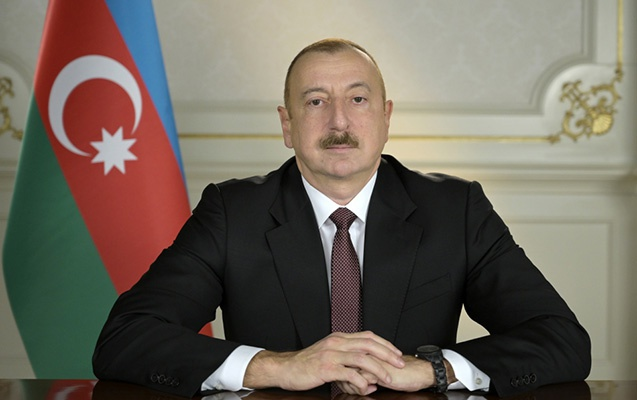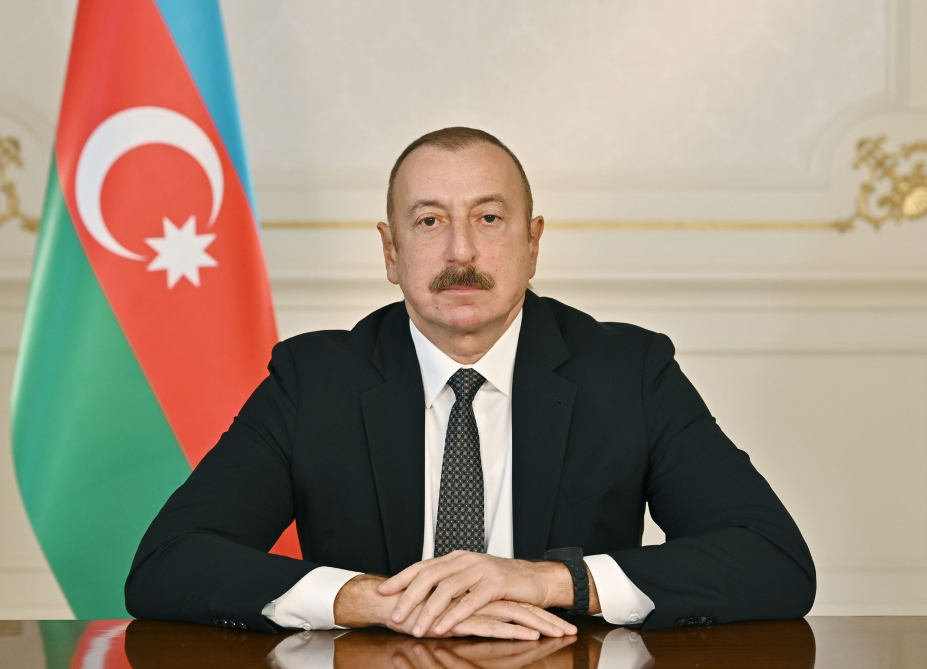Azərbaycan Respublikası Elm və Təhsil Nazirliyi
Riyaziyyat və Mexanika İnstitutu

## Qarabağ xəbərləri### Azərbaycan Respublikasında 2023-cü ilin “Heydər Əliyev İli” elan edilməsi haqqında Azərbaycan Respublikası Prezidentinin Sərəncamı### Azərbaycan Respublikasında 2022-ci ilin “Şuşa İli” elan edilməsi haqqında Azərbaycan Respublikası Prezidentinin Sərəncamı## Transactions 2006/4/XXVI

 Year: 2006 Volume: 4 Number: XXVI MATHEMATICS Akhundov A. Ya. Abstract [PDF] Determination of coefficient in the right hand side of a system of parabolic equations [PDF] 5 Akhmedov M.A., Ozkan O. Abstract [PDF] On application of differential transformations to N-dimensional problems for differential equations on a grid [PDF] 13 Aliev A.B., Kazimov A.A. Abstract [PDF] The asymptotic behavior of weak solution of Cauchy problem for a class Sobolev type semilinear equation [PDF] 23 Aliyev Ya.N. Abstract [PDF] On the basis properties of root functions of Sturm-Liouville problems with affine boundary conditions [PDF] 31 Amirov R.Kh. Abstract [PDF] Transformation operator for Sturm-Liouville operators with singularity and discontinuity conditions inside an interval [PDF] 35 Amirova L.I. Abstract [PDF] On solvability of a class of fourth order operator-differential equations[PDF] 55 Bagirov Sh.G. Abstract [PDF] On asymptotics of periodical in time solutions of second order nonlinear parabolic equations in cylindrical domains [PDF] 63 Bilalov B.T. Abstract [PDF] On operator calculus and spectral representation in Banach spaces[PDF] 75 Gadjiev A.G., Ragimov F.G. Abstract [PDF] On generalization of a class of the first passage time of random walk for the linear boundary [PDF] 89 Gasimov Z.A. Abstract [PDF] On a generalization of a system of exponents in weight spaces[PDF] 97 Gasymov T.B., Mammadova Sh.J. Abstract [PDF] On convergence of spectral expansions for one discontinuous problem with spectral parameter in the boundary condition [PDF] 103 Khalilov E.H. Abstract [PDF] On approximate solution of one class of two-dimensional singular integral equations[PDF] 117 Mamedov E.N. Abstract [PDF] On completeness of a part of eigen and adjoined elements of second order operator bundles [PDF] 125 Mamedov Sh.G. Abstract [PDF] On dependence of equiconvergence rate on the module of continuity of coefficient P2 (x) of a fourth order differential operator [PDF] 131 Mamedov Yu.A., Akhmedov S.Z. Abstract [PDF] On solution of a mixed problem for an equation of the fourth order with discontinuous coefficient[PDF] 137 Mammadzada (Mirzoyeva) K.S. Abstract [PDF] Behavior of solution of the second order quasilinear parabolic equation in unbounded domain [PDF] 145 Mirzoyev S.S., Aliyev A.R. Abstract [PDF] Initial boundary value problems for a class of third order operator-differential equations with variable coefficients[PDF] 153 Namazov F.A. Abstract [PDF] The inverse scattering problem for a system of first order ordinary differential equations on a semi-axis with the same incident waves [PDF] 165 Suleymanov N.A. Abstract [PDF] Initial boundary-value problem for a class of quasilinear Sobolev type equations [PDF] 173 Yusufov V. Sh. Abstract [PDF] Partially ordered systems of open sets semigroups of open mappings [PDF] 183 MECHANICS Agaev H.N. Abstract [PDF] Stress state of nonlinearly viscoelastic annular disk of variable thickness at rotation [PDF] 189 Najafov M.A. Abstract [PDF] Aeroelastic vibrations and stability of a conical shell streamlined by gas flow with high supersonic speed [PDF] 195 Suleymanov N.A. Abstract [PDF] On a frequency response of a pre-strained many-layered slab on a rigid foundation [PDF] 203 APPLIED PROBLEMS OF MATHEMATICS AND MECHANICS Akhmedov N.K. Abstract [PDF] Torsion of radilly laminated sphere of small thickness[PDF] 213 Mekhtiev M.F., Gyulmamedov M.K. Abstract [PDF] Forced vibrations of a spherical shell when tangential displacements and radial strains don’t exist on its face [PDF] 225 Panakhov G.M., Bakhtiyarov S.I., Shakhverdiyev A.Kh., Abbasov E.M. Abstract [PDF] Kinetics of gas generation in water solution[PDF] 239
Azərbaycanda COVID-19 ilə bağlı statistika
• Virusa yoluxan

832809

• Sağalan

822263

• Yeni yoluxan

0

• Aktiv xəstə

225

• Ölüm halı

10321

• Test edilib

7,684,217